# MPI topic: Data types

##### Experimental html version of Parallel Programming in MPI, OpenMP, and PETSc by Victor Eijkhout. download the textbook at https:/theartofhpc.com/pcse
$\newcommand\inv{^{-1}}\newcommand\invt{^{-t}} \newcommand\bbP{\mathbb{P}} \newcommand\bbR{\mathbb{R}} \newcommand\defined{ \mathrel{\lower 5pt \hbox{{\equiv\atop\mathrm{D}}}}}$ 6.1 : The \texttt{MPI\_Datatype} data type
6.2 : Predefined data types
6.2.1 : C/C++
6.2.2 : Fortran
6.2.2.1 : Fortran90 kind-defined types
6.2.3 : Python
6.2.3.1 : Type correspondences MPI / Python
6.2.5 : Matching language type to MPI type
6.2.5.1 : Type matching in C
6.2.5.2 : Type matching in Fortran
6.3 : Derived datatypes
6.3.1 : Basic calls
6.3.1.1 : Create calls
6.3.1.2 : Commit and free
6.3.2 : Contiguous type
6.3.3 : Vector type
6.3.3.1 : Two-dimensional arrays
6.3.4 : Subarray type
6.3.5 : Distributed array type
6.3.6 : Indexed type
6.3.7 : Struct type
6.4 : Big data types
6.4.1 : C
6.4.2 : Fortran
6.4.3 : Count datatype
6.4.4 : MPI 3 temporary solution
6.5 : Type maps and type matching
6.6 : Type extent
6.6.1 : Extent and true extent
6.6.2 : Extent resizing
6.6.2.1 : Example 1
6.6.2.2 : Example 2
6.6.2.3 : Example: dynamic vectors
6.6.2.4 : Example: transpose
6.7 : Reconstructing types
6.8 : Packing
6.9 : Review questions

# 6 MPI topic: Data types

In the examples you have seen so far, every time data was sent it was as a contiguous buffer with elements of a single type. In practice you may want to send noncontiguous or heterogeneous data.

• As an example of noncontiguous data, communicating the real parts of an array of complex numbers means specifying every other number.
• Heterogeneous data is needed when communicating a C structure or Fortran type with more than one type of element.
The datatypes you have dealt with so far are known as predefined datatype s; the datatypes you create to deal with other data are known as derived datatype s.

## 6.1 The \texttt{MPI\_Datatype} data type

crumb trail: > mpi-data > The \texttt{MPI\_Datatype} data type

Datatypes such as MPI_INT are values of the type MPI_Datatype  . This type is handled differently in different languages.

In C you can declare variables as

MPI_Datatype mytype;


Fortran note In Fortran before 2008, datatypes variables are stored in Integer variables. With the Fortran2008 standard, datatypes are Fortran derived types:

!! vector.F90
Type(MPI_Datatype) :: newvectortype

Implementationwise speaking, these types have exactly one member, MPI_VAL  , which is the same integer as was used for that datatype in the earlier Fortran version. End of Fortran note

Python note There is a class

mpi4py.MPI.Datatype

with predefined values such as
mpi4py.MPI.Datatype.DOUBLE

which are themselves objects with methods for creating derived types; see section  6.3.1  .

MPL note MPL mostly handles datatypes through subclasses of the layout class. Layouts are MPL routines are templated over the data type.

// sendlong.cxx
mpl::contiguous_layout<long long> v_layout(v.size());
comm.send(v.data(), v_layout, 1); // send to rank 1

Also works with complex of float and double.

The data types, where MPL can infer their internal representation, are enumeration types, C arrays of constant size and the template classes std::array , std::pair and std::tuple of the C++ Standard Template Library. The only limitation is, that the C array and the mentioned template classes hold data elements of types that can be sent or received by MPL. End of MPL note

MPL note Should you need the MPI_Datatype object contained in an MPL layout, there is an access function native_handle. End of MPL note

## 6.2 Predefined data types

crumb trail: > mpi-data > Predefined data types

MPI has a number of predefined data types of various kinds

• First of all there are the types corresponding to the simple data types of the host languages. The names are made to resemble the types of C and Fortran, for instance MPI_FLOAT and MPI_DOUBLE corresponding to float and double in C, versus MPI_REAL and MPI_DOUBLE_PRECISION corresponding to \finline{Real} and \finline{Double precision} in Fortran.
• The types MPI_PACKED and MPI_BYTE do not correspond to language types.
• The type MPI_Aint (and the Fortran kind MPI_ADDRESS_KIND  ) is used in RMA windows; see section  9.3.1  .
• The type MPI_Offset (and the corresponding Fortran MPI_OFFSET_KIND kind) is used to define MPI_Offset quantities, used in file I/O; section  10.2.2  .
• The type MPI_Count describes buffers; see section  6.4  .
• The type MPI_CHAR corresponds to a character, which is not the same as a C char : it can be more than one byte. Also, MPI converts between native character representations when communicating between different architectures.

### 6.2.1 C/C++

crumb trail: > mpi-data > Predefined data types > C/C++

Here we illustrate for C/C++ the correspondence between a type used to declare a variable, and how this type appears in MPI communication routines:

long int i;
MPI_Send(&i,1,MPI_LONG,target,tag,comm);

See table  6.1  .

 \toprule C type MPI type \midrule char MPI_CHAR unsigned char MPI_UNSIGNED_CHAR char MPI_SIGNED_CHAR short MPI_SHORT unsigned short MPI_UNSIGNED_SHORT int MPI_INT unsigned int MPI_UNSIGNED long int MPI_LONG unsigned long int MPI_UNSIGNED_LONG long long int MPI_LONG_LONG_INT float MPI_FLOAT double MPI_DOUBLE long double MPI_LONG_DOUBLE unsigned char MPI_BYTE (does not correspond to a C type) MPI_PACKED \bottomrule

TABULAR 6.1: Predefined datatypes in C

 \toprule C9 type MPI type \midrule _Bool MPI_C_BOOL float _Complex MPI_C_COMPLEX MPI_C_FLOAT_COMPLEX double _Complex MPI_C_DOUBLE_COMPLEX long double _Complex MPI_C_LONG_DOUBLE_COMPLEX \bottomrule

TABULAR 6.2: C99 synonym types.

 \toprule C11 type MPI type \midrule int8_t MPI_INT8_T int16_t MPI_INT16_T int32_t MPI_INT32_T int64_t MPI_INT64_T \midrule uint8_t MPI_UINT8_T uint16_t MPI_UINT16_T uint32_t MPI_UINT32_T uint64_t MPI_UINT64_T \bottomrule

TABULAR 6.3: C11 fixed width integer types.

• There is some, but not complete, support for C99 types; see table  6.2  .
• There is support for C11 fixed width integer types; see table  6.3  .
• The MPI_LONG_INT type is not an integer type, but rather a long and an int packed together; see section  3.10.1.1  .
• See section  6.2.4 for MPI_Aint and more about byte counting.

### 6.2.2 Fortran

crumb trail: > mpi-data > Predefined data types > Fortran

\hbox{

 \toprule MPI_CHARACTER Character(Len=1) MPI_INTEGER MPI_REAL MPI_DOUBLE_PRECISION MPI_COMPLEX MPI_LOGICAL MPI_BYTE MPI_PACKED \bottomrule

 \toprule MPI_INTEGER1 MPI_INTEGER2 MPI_INTEGER4 MPI_INTEGER8 MPI_INTEGER16 MPI_REAL2 MPI_REAL4 MPI_REAL8 MPI_DOUBLE_COMPLEX \ \kern20pt Complex(Kind=Kind(0.d0)) \bottomrule

}

TABLE 6.4: Standard Fortran types (left) and common extension (right)

Table  6.4 lists standard Fortran types and common extensions. Not all the types in the right table need be supported; for instance MPI_INTEGER16 may not exist, in which case it will be equivalent to MPI_DATATYPE_NULL  .

The default integer type MPI_INTEGER is equivalent to INTEGER(KIND=MPI_INTEGER_KIND)  .

#### 6.2.2.1 Fortran90 kind-defined types

crumb trail: > mpi-data > Predefined data types > Fortran > Fortran90 kind-defined types

If your Fortran90 code uses KIND to define scalar types with specified precision, you need to use the following routines to make MPI equivalences of Fortran scalar types :

• MPI_Type_create_f90_integer
• MPI_Type_create_f90_real
• MPI_Type_create_f90_complex  .

Example of an integer kind;

INTEGER ( KIND = SELECTED_INT_KIND(15) ) , &
DIMENSION(100) :: array
INTEGER :: root , error
Type(MPI_Datatype) :: integertype

CALL MPI_Type_create_f90_integer( 15 , integertype , error )
CALL MPI_Bcast ( array , 100 , &
integertype , root , MPI_COMM_WORLD , error )
! error parameter optional in f08, both routines.


\fsnippetwithoutput{kindsend}{examples/mpi/f08}{kindsend}

Example of a real kind:

REAL ( KIND = SELECTED_REAL_KIND(15 ,300) ) , &
DIMENSION(100) :: array
CALL MPI_Type_create_f90_real( 15 , 300 , realtype , error )


Example of a complex kind:

COMPLEX ( KIND = SELECTED_REAL_KIND(15 ,300) ) , &
DIMENSION(100) :: array
CALL MPI_Type_create_f90_complex( 15 , 300 , complextype , error )


Remark The MPI types thus created are predefined data types, so there is no need to commit or free them.
End of remark

### 6.2.3 Python

crumb trail: > mpi-data > Predefined data types > Python

Python note This section  6.2.3 discusses of predefined datatypes in Python.

In python, all buffer data comes from Numpy  .

 \toprule mpi4py type NumPy type \midrule MPI.INT np.intc np.int32 MPI.LONG np.int64 MPI.FLOAT np.float32 MPI.DOUBLE np.float64 \bottomrule

In this table we see that Numpy has three integer types, one corresponding to C  int s, and two with the number of bits explicitly indicated. There used to be a np.int type, but this is deprecated as of Numpy 1.20  .

Examples:

\psnippetwithoutput{npintc}{examples/mpi/p}{intsize}

## allgatherv.py
mycount = procid+1
my_array = np.empty(mycount,dtype=np.float64)


#### 6.2.3.1 Type correspondences MPI / Python

crumb trail: > mpi-data > Predefined data types > Python > Type correspondences MPI / Python

Above we saw that the number of bytes of a Numpy type can be deduced from

sizeofint = np.dtype('intc').itemsize


It is possible to derive the Numpy type corresponding to an MPI type:

## typesize.py
datatype = MPI.FLOAT
typecode = MPI._typecode(datatype)
assert typecode is not None # check MPI datatype is built-in
dtype = np.dtype(typecode)


crumb trail: > mpi-data > Predefined data types > Byte addressing types

So far we have mostly been taking about datatypes in the context of sending them. The MPI_Aint type is not so much for sending, as it is for describing the size of objects, such as the size of an MPI_Win object (section  9.1  ) or byte displacements in MPI_Type_create_hindexed  .

Addresses have type MPI_Aint  . The start of the address range is given in MPI_BOTTOM  . See also the MPI_Sizeof (section  6.2.5  ) and MPI_Get_address (section  6.3.6  ) routines.

Variables of type MPI_Aint can be sent as MPI_AINT :

MPI_Aint address;

See section  9.5.2 for an example.

In order to prevent overflow errors in byte calculations there are support routines MPI_Aint_add

MPI_Aint MPI_Aint_add(MPI_Aint base, MPI_Aint disp)

and similarly MPI_Aint_diff  .

Fortran note The equivalent of MPI_Aint in Fortran \indexmpishowf{MPI_Aint} in Fortran is an integer of kind MPI_ADDRESS_KIND :

integer(kind=MPI_ADDRESS_KIND) :: winsize


Using this integer kind to compute the size of a window also requires being able to query the size of the datatype in that window. See section  6.2.5 for details.

Example usage in MPI_Win_create :

call MPI_Sizeof(windowdata,window_element_size,ierr)
window_size = window_element_size*500
call MPI_Win_create( windowdata,window_size,window_element_size,... )

End of Fortran note

Python note Here is a good way for finding the size of numpy datatypes in bytes:

## putfence.py
intsize = np.dtype('int').itemsize
window_data = np.zeros(2,dtype=int)
win = MPI.Win.Create(window_data,intsize,comm=comm)


### 6.2.5 Matching language type to MPI type

crumb trail: > mpi-data > Predefined data types > Matching language type to MPI type

In some circumstances you may want to find the MPI type that corresponds to a type in your programming language.

• In C++ functions and classes can be templated, meaning that the type is not fully known:
template<typename T> {
class something<T> {
public:
void dosend(T input) {
MPI_Send( &input,1,/* ????? */ );
};
};

(Note that in MPL this is hardly ever needed because MPI calls are templated there.)
• Petsc installations use a generic identifier PetscScalar (or PetscReal  ) with a configuration-dependent realization.
• The size of a datatype is not always statically known, for instance if the Fortran KIND keyword is used.

Here are some MPI mechanisms that address this problem.

#### 6.2.5.1 Type matching in C

Datatypes in C can be translated to MPI types with MPI_Type_match_size where the typeclass argument is one of MPI_TYPECLASS_REAL  , MPI_TYPECLASS_INTEGER  , MPI_TYPECLASS_COMPLEX  .

\csnippetwithoutput{typematchc}{examples/mpi/c}{typematch}

The space that MPI takes for a structure type can be queried in a variety of ways. First of all MPI_Type_size counts the datatype size number of bytes occupied by the data in a type. That means that in an MPI vector datatype count the gaps.

// typesize.c
MPI_Type_vector(count,bs,stride,MPI_DOUBLE,&newtype);
MPI_Type_commit(&newtype);
MPI_Type_size(newtype,&size);
ASSERT( size==(count*bs)*sizeof(double) );


#### 6.2.5.2 Type matching in Fortran

In Fortran, the size of the datatype in the language can be obtained with MPI_Sizeof (note the nonoptional error parameter!). This routine is deprecated in MPI-4: use of storage_size and/or c_sizeof is recommended.

!! matchkind.F90
call MPI_Sizeof(x10,s10,ierr)
call MPI_Type_match_size(MPI_TYPECLASS_REAL,s10,mpi_x10)
call MPI_Type_size(mpi_x10,s10)
print *,"10 positions supported, MPI type size is",s10


Petsc has its own translation mechanism; see section  32.2  .

## 6.3 Derived datatypes

crumb trail: > mpi-data > Derived datatypes

MPI allows you to create your own data types, somewhat (but not completely\ldots) analogous to defining structures in a programming language. MPI data types are mostly of use if you want to send multiple items in one message.

There are two problems with using only predefined datatypes as you have seen so far.

• MPI communication routines can only send multiples of a single data type: it is not possible to send items of different types, even if they are contiguous in memory. It would be possible to use the MPI_BYTE data type, but this is not advisable.
• It is also ordinarily not possible to send items of one type if they are not contiguous in memory. You could of course send a contiguous memory area that contains the items you want to send, but that is wasteful of bandwidth, and of memory space on the receiving side.
With MPI data types you can solve these problems in several ways.
• You can create a new contiguous data type consisting of an array of elements of another data type. There is no essential difference between sending one element of such a type and multiple elements of the component type.
• You can create a vector data type consisting of regularly spaced blocks of elements of a component type. This is a first solution to the problem of sending noncontiguous data.
• For not regularly spaced data, there is the indexed data type  , where you specify an array of index locations for blocks of elements of a component type. The blocks can each be of a different size.
• The struct data type can accomodate multiple data types.
And you can combine these mechanisms to get irregularly spaced heterogeneous data, et cetera.

### 6.3.1 Basic calls

crumb trail: > mpi-data > Derived datatypes > Basic calls

The typical sequence of calls for creating a new datatype is as follows:

• You need a variable for the datatype; this is of type MPI_Datatype  .
• There is a create call, followed by a commit' call where MPI performs internal bookkeeping and optimizations; we will discuss this in great detail below.
• The type needs to be committed'. After this:
• The datatype is used, possibly multiple times;
• When the datatype is no longer needed, it must be freed to prevent memory leaks; see section  6.3.1.2  .
In code:
MPI_Datatype newtype;
MPI_Type_something( < oldtype specifications >, &newtype );
MPI_Type_commit( &newtype );
/* code that uses your new type */
MPI_Type_free( &newtype );


In Fortran2008:

Type(MPI_Datatype) :: newvectortype
call MPI_Type_something( <oldtype specification>, &
newvectortype)
call MPI_Type_commit(newvectortype)
!! code that uses your type
call MPI_Type_free(newvectortype)


Python note The various type creation routines are methods of the datatype classes, after which commit and free are methods on the new type.

## vector.py
source = np.empty(stride*count,dtype=np.float64)
target = np.empty(count,dtype=np.float64)
if procid==sender:
newvectortype = MPI.DOUBLE.Create_vector(count,1,stride)
newvectortype.Commit()
comm.Send([source,1,newvectortype],dest=the_other)
newvectortype.Free()
comm.Recv([target,count,MPI.DOUBLE],source=the_other)


MPL note In MPL type creation routines are in the main namespace, templated over the datatypes.

// vector.cxx
vector<double>
source(stride*count);
if (procno==sender) {
mpl::strided_vector_layout<double>
newvectortype(count,1,stride);
comm_world.send
(source.data(),newvectortype,the_other);
}

The commit call is part of the type creation, and freeing is done in the destructor. End of MPL note

#### 6.3.1.1 Create calls

crumb trail: > mpi-data > Derived datatypes > Basic calls > Create calls

The MPI_Datatype variable gets its value by a call to one of the following routines:

• MPI_Type_contiguous for contiguous blocks of data; section  6.3.2 ;
• MPI_Type_vector for regularly strided data; section  6.3.3 ;
• MPI_Type_create_subarray for subsets out higher dimensional block; section  6.3.4 ;
• MPI_Type_create_struct for heterogeneous irregular data; section  6.3.7 ;
• MPI_Type_indexed and MPI_Type_hindexed for irregularly strided data; section  6.3.6  .
These calls take an existing type, whether predefined or also derived, and produce a new type.

#### 6.3.1.2 Commit and free

crumb trail: > mpi-data > Derived datatypes > Basic calls > Commit and free

It is necessary to call MPI_Type_commit on a new data type, which makes MPI do the indexing calculations for the data type.

When you no longer need the data type, you call MPI_Type_free  . (This is typically not needed in OO APIs  .) This has the following effects:

• The definition of the datatype identifier will be changed to MPI_DATATYPE_NULL  .
• Any communication using this data type, that was already started, will be completed succesfully.
• Datatypes that are defined in terms of this data type will still be usable.

### 6.3.2 Contiguous type

crumb trail: > mpi-data > Derived datatypes > Contiguous type

The simplest derived type is the contiguous' type, constructed with MPI_Type_contiguous  .

A contigous type describes an array of items of an predefined or earlier defined type. There is no difference between sending one item of a contiguous type and multiple items of the constituent type.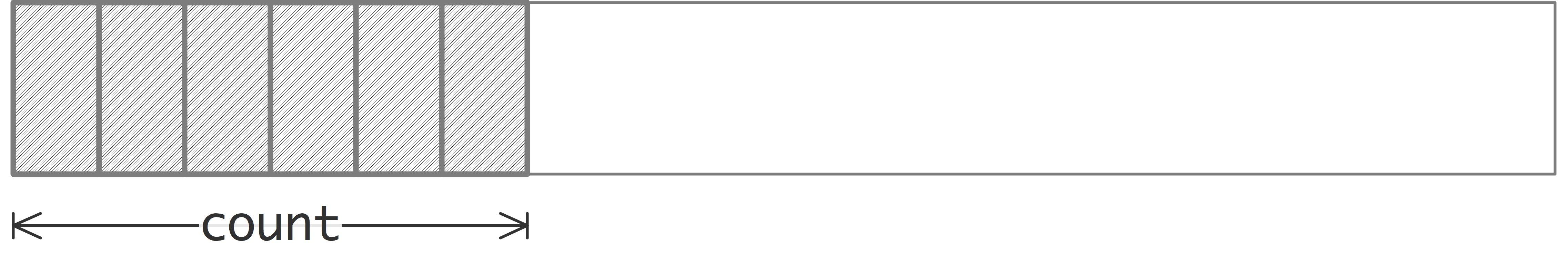FIGURE 6.5: A contiguous datatype is built up out of elements of a constituent type

This is illustrated in figure  6.5  .

// contiguous.c
MPI_Datatype newvectortype;
if (procno==sender) {
MPI_Type_contiguous(count,MPI_DOUBLE,&newvectortype);
MPI_Type_commit(&newvectortype);
MPI_Type_free(&newvectortype);
MPI_Status recv_status;
int recv_count;
MPI_Recv(target,count,MPI_DOUBLE,sender,0,comm,
&recv_status);
MPI_Get_count(&recv_status,MPI_DOUBLE,&recv_count);
ASSERT(count==recv_count);
}


!! contiguous.F90
integer :: newvectortype
if (mytid==sender) then
call MPI_Type_contiguous(count,MPI_DOUBLE_PRECISION,newvectortype)
call MPI_Type_commit(newvectortype)
call MPI_Type_free(newvectortype)
call MPI_Recv(target,count,MPI_DOUBLE_PRECISION,sender,0,comm,&
recv_status)
call MPI_Get_count(recv_status,MPI_DOUBLE_PRECISION,recv_count)
!ASSERT(count==recv_count);
end if


## contiguous.py
source = np.empty(count,dtype=np.float64)
target = np.empty(count,dtype=np.float64)
if procid==sender:
newcontiguoustype = MPI.DOUBLE.Create_contiguous(count)
newcontiguoustype.Commit()
comm.Send([source,1,newcontiguoustype],dest=the_other)
newcontiguoustype.Free()
comm.Recv([target,count,MPI.DOUBLE],source=the_other)


MPL note The MPL interface makes extensive use of contiguous_layout, as it is the main way to declare a nonscalar buffer; see note  3.2.4  . End of MPL note

MPL note Contiguous layouts can only use predefined types or other contiguous layouts as their old' type. To make a contiguous type for other layouts, use vector_layout :

// contiguous.cxx
mpl::contiguous_layout<int> type1(7);
mpl::vector_layout<int> type2(8,type1);

(Contrast this with strided_vector_layout; note  6.6  .) End of MPL note

### 6.3.3 Vector type

crumb trail: > mpi-data > Derived datatypes > Vector type

The simplest noncontiguous datatype is the vector' type, constructed with MPI_Type_vector  .

A vector type describes a series of blocks, all of equal size, spaced with a constant stride.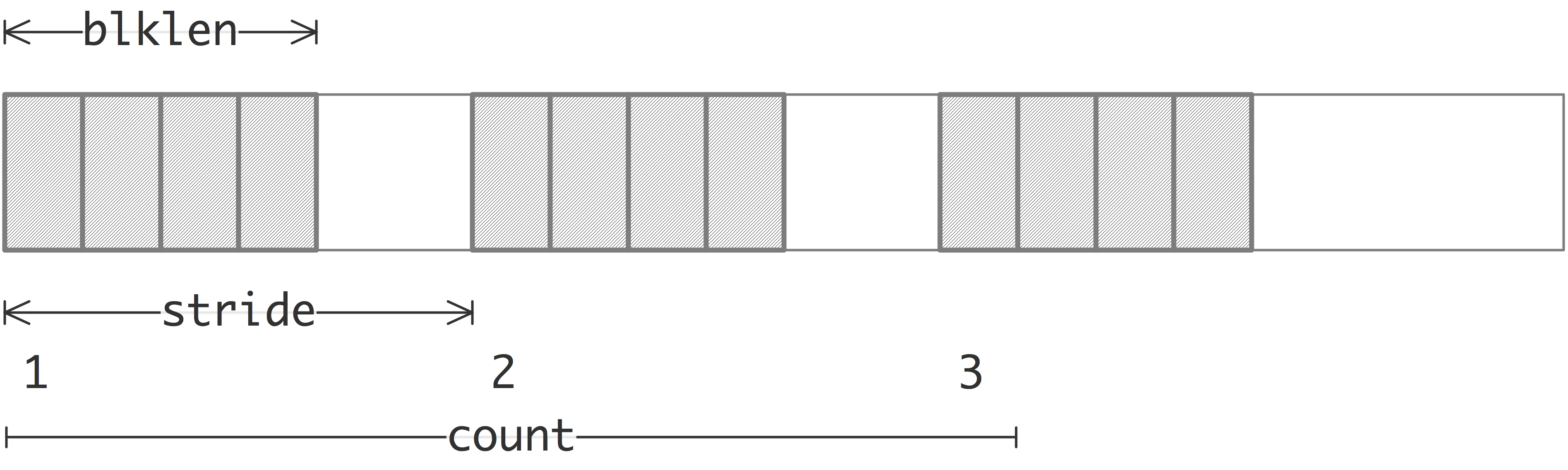FIGURE 6.6: A vector datatype is built up out of strided blocks of elements of a constituent type

This is illustrated in figure  6.6  .

The vector datatype gives the first nontrivial illustration that receiver}. If the sender sends b  blocks of length  l each, the receiver can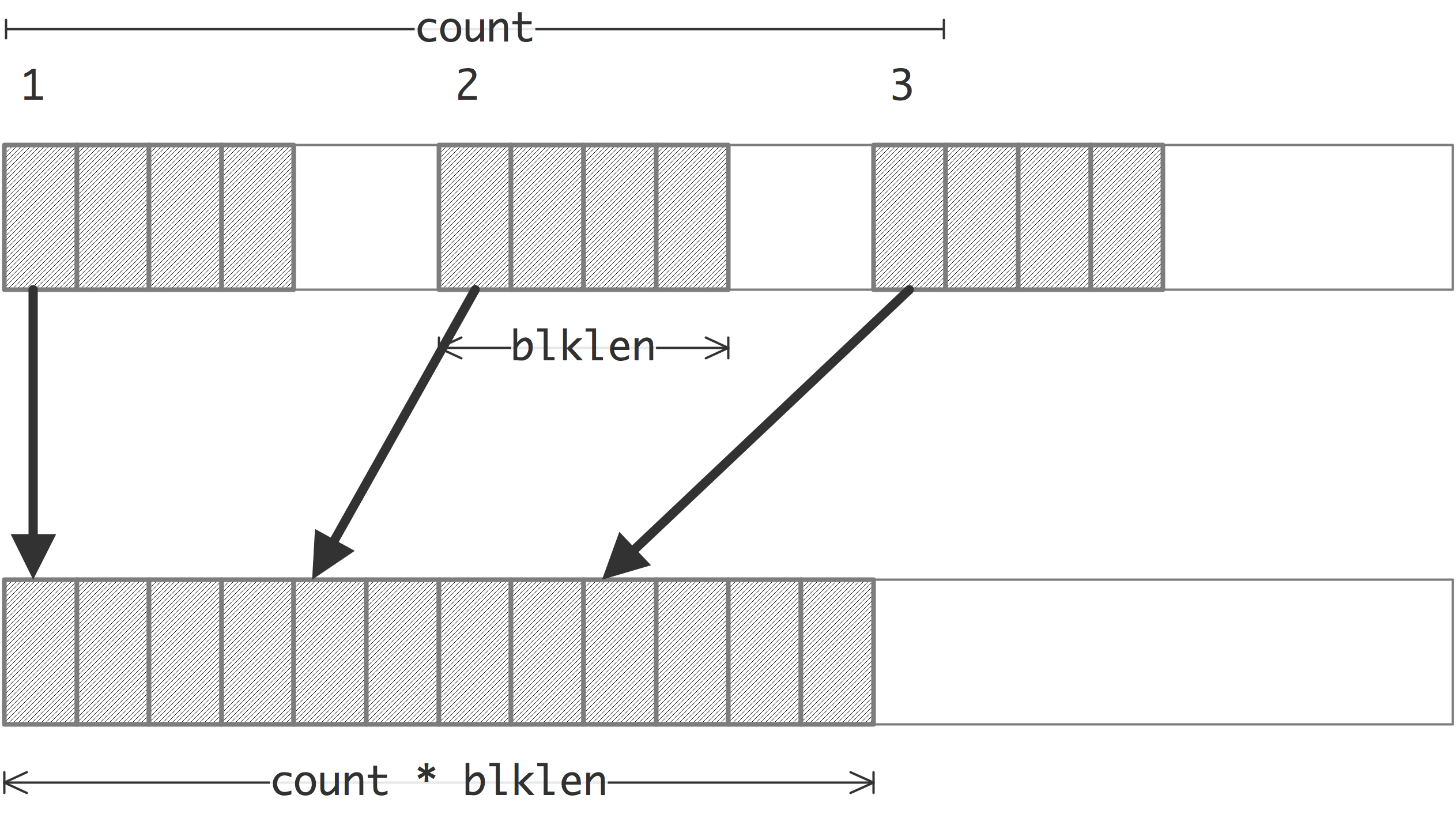\caption{Sending a vector datatype and receiving it as predefined or contiguous}

receive them as bl contiguous elements, either as a contiguous datatype, or as a contiguous buffer of an predefined type; see figure  6.6  . The receiver has no knowledge of the stride of the datatype on the sender.

In this example a vector type is created only on the sender, in order to send a strided subset of an array; the receiver receives the data as a contiguous block.

// vector.c
source = (double*) malloc(stride*count*sizeof(double));
target = (double*) malloc(count*sizeof(double));
MPI_Datatype newvectortype;
if (procno==sender) {
MPI_Type_vector(count,1,stride,MPI_DOUBLE,&newvectortype);
MPI_Type_commit(&newvectortype);
MPI_Send(source,1,newvectortype,the_other,0,comm);
MPI_Type_free(&newvectortype);
MPI_Status recv_status;
int recv_count;
MPI_Recv(target,count,MPI_DOUBLE,the_other,0,comm,
&recv_status);
MPI_Get_count(&recv_status,MPI_DOUBLE,&recv_count);
ASSERT(recv_count==count);
}


We illustrate Fortran2008:

if (mytid==sender) then
call MPI_Type_vector(count,1,stride,MPI_DOUBLE_PRECISION,&
newvectortype)
call MPI_Type_commit(newvectortype)
call MPI_Type_free(newvectortype)
if ( .not. newvectortype==MPI_DATATYPE_NULL) then
print *,"Trouble freeing datatype"
else
print *,"Datatype successfully freed"
end if
call MPI_Recv(target,count,MPI_DOUBLE_PRECISION,sender,0,comm,&
recv_status)
call MPI_Get_count(recv_status,MPI_DOUBLE_PRECISION,recv_count)
end if


In legacy mode Fortran90, code stays the same except that the type is declared as Integer :

!! vector.F90
integer :: newvectortype
integer :: recv_count
call MPI_Type_vector(count,1,stride,MPI_DOUBLE_PRECISION,&
newvectortype,err)
call MPI_Type_commit(newvectortype,err)


Python note The vector creation routine is a method of the datatype class. For the general discussion, see section  6.3.1  .

## vector.py
source = np.empty(stride*count,dtype=np.float64)
target = np.empty(count,dtype=np.float64)
if procid==sender:
newvectortype = MPI.DOUBLE.Create_vector(count,1,stride)
newvectortype.Commit()
comm.Send([source,1,newvectortype],dest=the_other)
newvectortype.Free()
comm.Recv([target,count,MPI.DOUBLE],source=the_other)


MPL note MPL has the strided_vector_layout class as equivalent of the vector type:

// vector.cxx
vector<double>
source(stride*count);
if (procno==sender) {
mpl::strided_vector_layout<double>
newvectortype(count,1,stride);
comm_world.send
(source.data(),newvectortype,the_other);
}


(See note  6.5 for nonstrided vectors.) End of MPL note

#### 6.3.3.1 Two-dimensional arrays

crumb trail: > mpi-data > Derived datatypes > Vector type > Two-dimensional arrays

Figure  6.7 indicates one source of irregular data: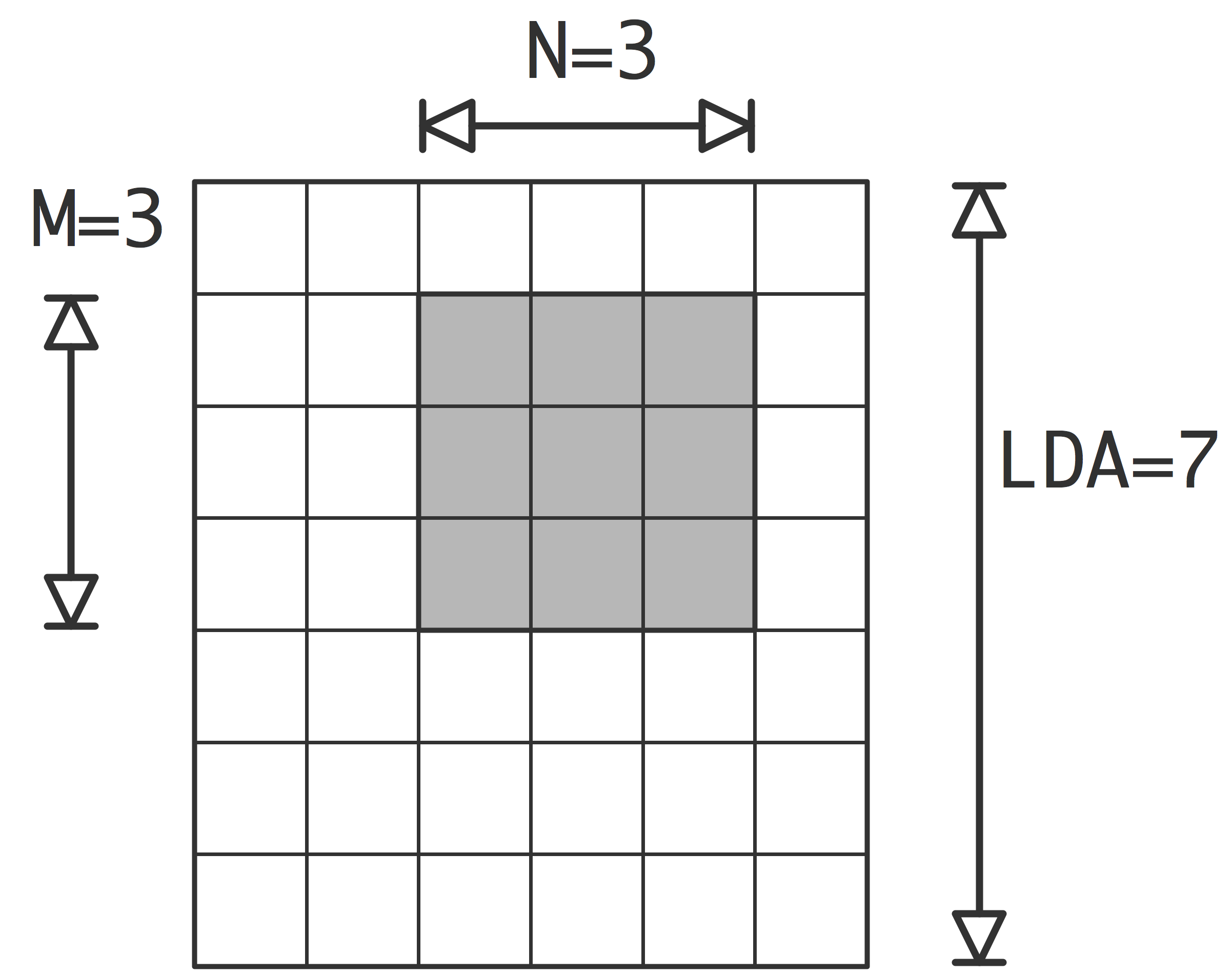FIGURE 6.7: Memory layout of a row and column of a matrix in column-major storage

with a matrix on column-major storage  , a column is stored in contiguous memory. However, a row of such a matrix is not contiguous; its elements being separated by a stride equal to the column length.

Exercise How would you describe the memory layout of a submatrix, if the whole matrix has size $M\times N$ and the submatrix $m\times n$?
End of exercise

As an example of this datatype, consider the example of transposing a matrix, for instance to convert between C and Fortran arrays. Suppose that a processor has a matrix stored in C, row-major, layout, and it needs to send a column to another processor. If the matrix is declared as

  int M,N; double mat[M][N]

then a column has $M$ blocks of one element, spaced $N$ locations apart. In other words:
MPI_Datatype MPI_column;
MPI_Type_vector(
/* count= */ M, /* blocklength= */ 1, /* stride= */ N,
MPI_DOUBLE, &MPI_column );

Sending the first column is easy:
MPI_Send( mat, 1,MPI_column, ... );

The second column is just a little trickier: you now need to pick out elements with the same stride, but starting at A  .
MPI_Send( &(mat), 1,MPI_column, ... );

You can make this marginally more efficient (and harder to read) by replacing the index expression by mat+1  .

Exercise Suppose you have a matrix of size $4N\times 4N$, and you want to send the elements A[4*i][4*j] with $i,j=0,\ldots,N-1$. How would you send these elements with a single transfer?
End of exercise

Exercise Allocate a matrix on processor zero, using Fortran column-major storage. Using $P$ sendrecv calls, distribute the rows of this matrix among the processors.
End of exercise

Python note In C and Fortran it's easy to apply a derived type to data in the middle of an array, for instance to extract an arbitrary column out of a C matrix, or row out of a Fortran matrix. While Python has no trouble describing sections from an array, usually it copies these instead of taking the address. Therefore, it is necessary to convert the matrix to a buffer and compute an explicit offset in bytes:

## rowcol.py
rowsize = 4; colsize = 5
coltype = MPI.INT.Create_vector(4, 1, 5)
coltype.Commit()
columntosend = 2
comm.Send\
( [np.frombuffer(matrix.data, intc,
offset=columntosend*np.dtype('intc').itemsize),
1,coltype],FIGURE 6.8: Send strided data from process zero to all others

Exercise Let processor 0 have an array $x$ of length $10P$, where $P$ is the number of processors. Elements $0,P,2P,\ldots,9P$ should go to processor zero, $1,P+1,2P+1,\ldots$ to processor 1, et cetera.

• Code this as a sequence of send/recv calls, using a vector datatype for the send, and a contiguous buffer for the receive.
• For simplicity, skip the send to/from zero. What is the most elegant solution if you want to include that case?
• For testing, define the array as $x[i]=i$.

(There is a skeleton code stridesend in the repository)
End of exercise

Exercise Write code to compare the time it takes to send a strided subset from an array: copy the elements by hand to a smaller buffer, or use a vector data type. What do you find? You may need to test on fairly large arrays.
End of exercise

### 6.3.4 Subarray type

crumb trail: > mpi-data > Derived datatypes > Subarray type

The vector datatype can be used for blocks in an array of dimension more than 2 by using it recursively. However, this gets tedious. Instead, there is an explicit subarray type MPI_Type_create_subarray  . This describes the dimensionality and extent of the array, and the starting point (the upper left corner') and extent of the subarray.

MPL note The templated subarray_layout class is constructed from a vector of triplets of global size / subblock size / first coordinate.

mpl::subarray_layout<int>(
{ {ny, ny_l, ny_0}, {nx, nx_l, nx_0} }
);

End of MPL note

Exercise Assume that your number of processors is $P=Q^3$, and that each process has an array of identical size. Use MPI_Type_create_subarray to gather all data onto a root process. Use a sequence of send and receive calls; MPI_Gather does not work here.

(There is a skeleton code cubegather in the repository)

End of exercise

Fortran note Subarrays are naturally supported in Fortran through array sections.

!! section.F90
integer,parameter :: siz=20
real,dimension(siz,siz) :: matrix = [ ((j+(i-1)*siz,i=1,siz),j=1,siz) ]
real,dimension(2,2) :: submatrix
if (procno==0) then
call MPI_Send(matrix(1:2,1:2),4,MPI_REAL,1,0,comm)
else if (procno==1) then
call MPI_Recv(submatrix,4,MPI_REAL,0,0,comm,MPI_STATUS_IGNORE)
if (submatrix(2,2)==22) then
print *,"Yay"
else
print *,"nay...."
end if
end if

However, there is a subtlety with non-blocking operations: for a non-contiguous buffer a temporary is created, which is released after the MPI call. This is correct for blocking sends, but for non-blocking the temporary has to stay around till the wait call.
!! sectionisend.F90
integer :: siz
real,dimension(:,:),allocatable :: matrix
real,dimension(2,2) :: submatrix
siz = 20
allocate( matrix(siz,siz) )
matrix = reshape( [ ((j+(i-1)*siz,i=1,siz),j=1,siz) ], (/siz,siz/) )
call MPI_Isend(matrix(1:2,1:2),4,MPI_REAL,1,0,comm,request)
call MPI_Wait(request,MPI_STATUS_IGNORE)
deallocate(matrix)

In MPI-3 the variable MPI_SUBARRAYS_SUPPORTED indicates support for this mechanism:
if ( .not. MPI_SUBARRAYS_SUPPORTED ) then
print *,"This code will not work"
call MPI_Abort(comm,0)
end if

End of Fortran note

The possibilities for the order parameter are MPI_ORDER_C and MPI_ORDER_FORTRAN  . However, this has nothing to do with the order of traversal of elements; it determines how the bounds of the subarray are interpreted. As an example, we fill a $4\times 4$ array in C order with the numbers $0\cdots15$, and send the $[0,1]\times [0\cdots4]$ slice two ways, first C order, then Fortran order: \cverbatimsnippet[code/mpi/c/row2col.c]{row2colsub}

The receiver receives the following, formatted to bring out where the numbers originate:

Received C order:
0.000 1.000 2.000 3.000
4.000 5.000 6.000 7.000
0.000 1.000
4.000 5.000
8.000 9.000
12.000 13.000


### 6.3.5 Distributed array type

crumb trail: > mpi-data > Derived datatypes > Distributed array type

Each dimension can independently be distributed as MPI_DISTRIBUTE_BLOCK  , MPI_DISTRIBUTE_CYCLIC  , MPI_DISTRIBUTE_NONE  ,

With the cyclic distribution, the amount of cyclicity can be indicated by setting dargs[id] to a certain number.

With the block distribution, blocks can be set explicitly in dargs[id]  , but MPI_DISTRIBUTE_DFLT_DARG causes an even distribution to be found.

Ordering can be MPI_ORDER_C or MPI_ORDER_FORTRAN  .

### 6.3.6 Indexed type

crumb trail: > mpi-data > Derived datatypes > Indexed type

The indexed datatype, constructed with MPI_Type_indexed can send arbitrarily located elements from an array of a single datatype. You need to supply an array of index locations, plus an array of blocklengths with a separate blocklength for each index. The total number of elements sent is the sum of the blocklengths.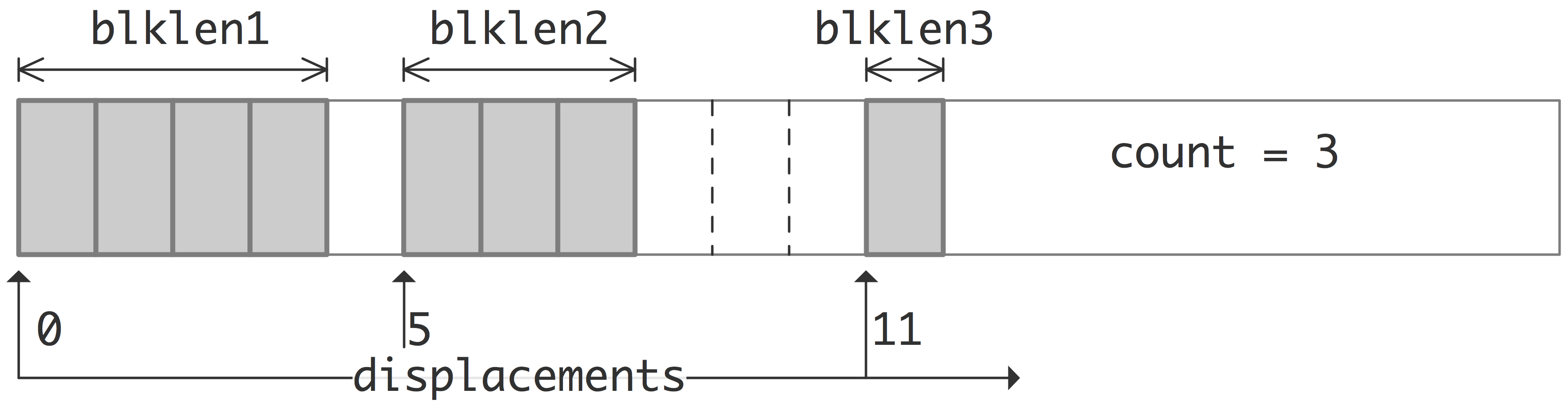FIGURE 6.9: The elements of an MPI Indexed datatype

The following example picks items that are on prime number-indexed locations.

// indexed.c
displacements = (int*) malloc(count*sizeof(int));
blocklengths = (int*) malloc(count*sizeof(int));
source = (int*) malloc(totalcount*sizeof(int));
target = (int*) malloc(targetbuffersize*sizeof(int));
MPI_Datatype newvectortype;
if (procno==sender) {
MPI_Type_indexed(count,blocklengths,displacements,MPI_INT,&newvectortype);
MPI_Type_commit(&newvectortype);
MPI_Send(source,1,newvectortype,the_other,0,comm);
MPI_Type_free(&newvectortype);
MPI_Status recv_status;
int recv_count;
MPI_Recv(target,targetbuffersize,MPI_INT,the_other,0,comm,
&recv_status);
MPI_Get_count(&recv_status,MPI_INT,&recv_count);
ASSERT(recv_count==count);
}

For Fortran we show the legacy syntax for once:
!! indexed.F90
integer :: newvectortype;
ALLOCATE(indices(count))
ALLOCATE(blocklengths(count))
ALLOCATE(source(totalcount))
ALLOCATE(targt(count))
if (mytid==sender) then
call MPI_Type_indexed(count,blocklengths,indices,MPI_INT,&
newvectortype,err)
call MPI_Type_commit(newvectortype,err)
call MPI_Type_free(newvectortype,err)
call MPI_Recv(targt,count,MPI_INT,sender,0,comm,&
recv_status,err)
call MPI_Get_count(recv_status,MPI_INT,recv_count,err)
!    ASSERT(recv_count==count);
end if

## indexed.py
displacements = np.empty(count,dtype=int)
blocklengths = np.empty(count,dtype=int)
source = np.empty(totalcount,dtype=np.float64)
target = np.empty(count,dtype=np.float64)
if procid==sender:
newindextype = MPI.DOUBLE.Create_indexed(blocklengths,displacements)
newindextype.Commit()
comm.Send([source,1,newindextype],dest=the_other)
newindextype.Free()
comm.Recv([target,count,MPI.DOUBLE],source=the_other)


MPL note In MPL  , the indexed_layout is based on a vector of 2-tuples denoting block length / block location.

// indexed.cxx
const int count = 5;
mpl::contiguous_layout<int>
fiveints(count);
mpl::indexed_layout<int>
indexed_where{ { {1,2}, {1,3}, {1,5}, {1,7}, {1,11} } };

if (procno==sender) {
auto recv_status =
comm_world.recv( target_buffer.data(),fiveints, sender );
int recv_count = recv_status.get_count<int>();
assert(recv_count==count);
}

End of MPL note

MPL note The size/displacement arrays for MPI_Gatherv  / MPI_Alltoallv are handled through a layouts object, which is basically a vector of layout objects.

mpl::layouts<int> receive_layout;
for ( int iproc=0,loc=0; iproc<nprocs; iproc++ ) {
auto siz = size_buffer.at(iproc);
( mpl::indexed_layout<int>( {{ siz,loc }} ) );
loc += siz;
}

End of MPL note

MPL note For the case where all block lengths are the same, use indexed_block_layout:

// indexedblock.cxx
mpl::indexed_block_layout<int>
indexed_where(  1, {2,3,5,7,11} );

End of MPL note

You can also MPI_Type_create_hindexed which describes blocks of a single old type, but with index locations in bytes, rather than in multiples of the old type.

int MPI_Type_create_hindexed
(int count, int blocklens[], MPI_Aint indices[],
MPI_Datatype old_type,MPI_Datatype *newtype)

A slightly simpler version, MPI_Type_create_hindexed_block assumes constant block length.

There is an important difference between the hindexed and the above MPI_Type_indexed : that one described offsets from a base location; these routines describes absolute memory addresses. You can use this to send for instance the elements of a linked list. You would traverse the list, recording the addresses of the elements with MPI_Get_address  . (The routine \indexmpidepr{MPI_Address} is deprecated.)

In C++ you can use this to send an std::  , that is, a vector object from the C++ standard library  , if the component type is a pointer.

### 6.3.7 Struct type

crumb trail: > mpi-data > Derived datatypes > Struct type

The structure type, created with MPI_Type_create_struct  , can contain multiple data types. (The routine MPI_Type_struct is deprecated with MPI-3.)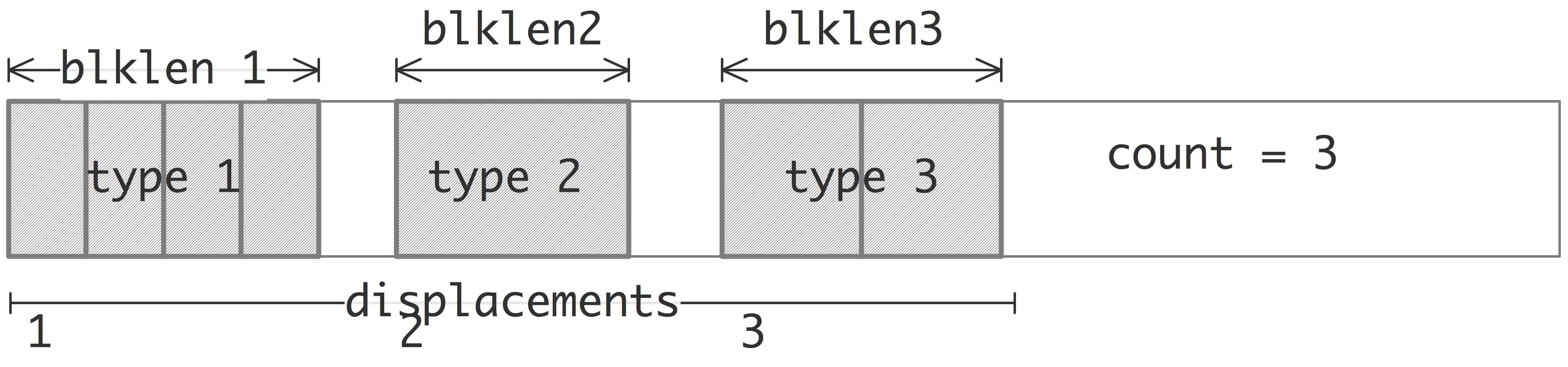FIGURE 6.10: The elements of an MPI Struct datatype

The specification contains a count' parameter that specifies how many blocks there are in a single structure. For instance,

struct {
int i;
float x,y;
} point;

has two blocks, one of a single integer, and one of two floats. This is illustrated in figure  6.10  .

• [ count  ] The number of blocks in this datatype. The blocklengths  , displacements  , types arguments have to be at least of this length.
• [ blocklengths  ] array containing the lengths of the blocks of each datatype.
• [ displacements  ] array describing the relative location of the blocks of each datatype.
• [ types  ] array containing the datatypes; each block in the new type is of a single datatype; there can be multiple blocks consisting of the same type.
In this example, unlike the previous ones, both sender and receiver create the structure type. With structures it is no longer possible to send as a derived type and receive as a array of a simple type. (It would be possible to send as one structure type and receive as another, as long as they have the same datatype signature  .)

// struct.c
struct object {
char c;
double x;
int i;
};
MPI_Datatype newstructuretype;
int structlen = 3;
int blocklengths[structlen]; MPI_Datatype types[structlen];
MPI_Aint displacements[structlen];

/*
* where are the components relative to the structure?
*/
MPI_Aint current_displacement=0;

// one character
blocklengths = 1; types = MPI_CHAR;
displacements = (size_t)&(myobject.c) - (size_t)&myobject;

// two doubles
blocklengths = 2; types = MPI_DOUBLE;
displacements = (size_t)&(myobject.x) - (size_t)&myobject;

// one int
blocklengths = 1; types = MPI_INT;
displacements = (size_t)&(myobject.i) - (size_t)&myobject;

MPI_Type_create_struct(structlen,blocklengths,displacements,types,&newstructuretype);
MPI_Type_commit(&newstructuretype);
if (procno==sender) {
MPI_Send(&myobject,1,newstructuretype,the_other,0,comm);
MPI_Recv(&myobject,1,newstructuretype,the_other,0,comm,MPI_STATUS_IGNORE);
}
MPI_Type_free(&newstructuretype);


Note the displacement calculations in this example, which involve some not so elegant pointer arithmetic. The following Fortran code uses MPI_Get_address  , which is more elegant, and in fact the only way address calculations can be done in Fortran.

!! struct.F90
Type object
character :: c
real*8,dimension(2) :: x
integer :: i
end type object
type(object) :: myobject
integer,parameter :: structlen = 3
type(MPI_Datatype) :: newstructuretype
integer,dimension(structlen) :: blocklengths
type(MPI_Datatype),dimension(structlen) :: types;
MPI_Aint,dimension(structlen) :: displacements
MPI_Aint :: base_displacement, next_displacement
if (procno==sender) then
myobject%c = 'x'
myobject%x(0) = 2.7; myobject%x(1) = 1.5
myobject%i = 37

!! component 1: one character
blocklengths(1) = 1; types(1) = MPI_CHAR
displacements(1) = next_displacement-base_displacement

!! component 2: two doubles
blocklengths(2) = 2; types(2) = MPI_DOUBLE
displacements(2) = next_displacement-base_displacement

!! component 3: one int
blocklengths(3) = 1; types(3) = MPI_INT
displacements(3) = next_displacement-base_displacement

if (procno==sender) then
call MPI_Recv(myobject,1,newstructuretype,sender,0,comm,MPI_STATUS_IGNORE)
end if
call MPI_Type_free(newstructuretype)


It would have been incorrect to write

displacement = 0;
displacement = displacement + sizeof(char);

since you do not know the way the compiler lays out the structure in memory\footnote{Homework question: what does the language standard say about this?}.

If you want to send more than one structure, you have to worry more about padding in the structure. You can solve this by adding an extra type MPI_UB for the upper bound' on the structure:

displacements = sizeof(myobject); types = MPI_UB;
MPI_Type_create_struct(struclen+1,.....);


MPL note One could describe the MPI struct type as a collection of displacements, to be applied to any set of items that conforms to the specifications. An MPL heterogeneous_layout on the other hand, incorporates the actual data. Thus you could write

// structscalar.cxx
char c; double x; int i;
if (procno==sender) {
c = 'x'; x = 2.4; i = 37; }
mpl::heterogeneous_layout object( c,x,i );
if (procno==sender)
comm_world.recv( mpl::absolute,object,sender );

Here, the absolute indicates the lack of an implicit buffer: the layout is absolute rather than a relative description. End of MPL note

MPL note More complicated data than scalars takes more work:

// struct.cxx
char c; vector<double> x(2); int i;
if (procno==sender) {
c = 'x'; x = 2.7; x = 1.5; i = 37; }
mpl::heterogeneous_layout object
( c,
mpl::make_absolute(x.data(),mpl::vector_layout<double>(2)),
i );
if (procno==sender) {
comm_world.recv( mpl::absolute,object,sender );
}

Note the make_absolute in addition to absolute mentioned above. End of MPL note

## 6.4 Big data types

crumb trail: > mpi-data > Big data types

The size parameter in MPI send and receive calls is of type integer, meaning that it's maximally (platform-dependent, but typically:) $2^{31}-1$. These day computers are big enough that this is a limitation. As of the MPI-4 standard, this has been solved by allowing a larger count parameter of type MPI_Count  . The implementation of this depends somewhat on the language.

\begin{mpifournote} {MPI Count type}

### 6.4.1 C

crumb trail: > mpi-data > Big data types > C

For every routine, such as MPI_Send with an integer count, there is a corresponding MPI_Send_c with a count of type MPI_Count  .

MPI_Count buffersize = 1000;
double *indata,*outdata;
indata = (double*) malloc( buffersize*sizeof(double) );
outdata = (double*) malloc( buffersize*sizeof(double) );
MPI_Allreduce_c(indata,outdata,buffersize,MPI_DOUBLE,MPI_SUM,MPI_COMM_WORLD);


{ \def\snippetcodefraction{.45} \def\snippetlistfraction{.5} \csnippetwithoutput{bigpingpong}{examples/mpi/c}{pingpongbig} }

### 6.4.2 Fortran

crumb trail: > mpi-data > Big data types > Fortran

The count parameter can be declared to be

use mpi_f08
Integer(kind=MPI_COUNT_KIND) :: count

Since Fortran has polymorphism, the same routine names can be used.

The legit way of coding:

!! typecheckkind.F90
integer(8) :: source,n=1
call MPI_Init()
call MPI_Send(source,n,MPI_INTEGER8, &
1,0,MPI_COMM_WORLD)


\ldots but you can see what's under the hood:

!! typecheck8.F90
integer(8) :: source,n=1
call MPI_Init()
call MPI_Send(source,n,MPI_INTEGER8, &
1,0,MPI_COMM_WORLD)


Routines using this type are not available unless using the mpi_f08 module. \end{mpifournote}

!! pingpongbig.F90
integer :: power,countbytes
Integer(KIND=MPI_COUNT_KIND) :: length
call MPI_Sizeof(length,countbytes,ierr)
if (procno==0) &
print *,"Bytes in count:",countbytes
length = 10**power
allocate( senddata(length),recvdata(length) )
call MPI_Send(senddata,length,MPI_DOUBLE_PRECISION, &
processB,0, comm)
call MPI_Recv(recvdata,length,MPI_DOUBLE_PRECISION, &
processB,0, comm,MPI_STATUS_IGNORE)


### 6.4.3 Count datatype

crumb trail: > mpi-data > Big data types > Count datatype

The MPI_Count datatype is defined as being large enough to accomodate values of

• the ordinary 4-byte integer type;
• the MPI_Aint type, sections 6.2.4 and  6.2.4 ;
• the MPI_Offset type, section  10.2.2  .

The size_t type in C/C++ is defined as big enough to contain the output of sizeof , that is, being big enough to measure any object.

### 6.4.4 MPI 3 temporary solution

crumb trail: > mpi-data > Big data types > MPI 3 temporary solution

Large messages were already possible by using derived types: to send a big data type of $10^{40}$ elements you would

• create a contiguous type with $10^{20}$ elements, and
• send $10^{20}$ elements of that type.
This often works, but it's not perfect. For instance, the routine MPI_Get_elements returns the total number of basic elements sent (as opposed to MPI_Get_count which would return the number of elements of the derived type). Since its output argument is of integer type, it can't store the right value.

The MPI-3 standard has addressed this through the introduction of an MPI_Count datatype, and new routines with an _x extension, that return that type of count. (In view of the embiggened' routines, this solution is no longer needed, and will probably be deprecated in later standards.)

Let us consider an example.

Allocating a buffer of more than 4Gbyte is not hard:

// vectorx.c
float *source=NULL,*target=NULL;
int mediumsize = 1<<30;
int nblocks = 8;
size_t datasize = (size_t)mediumsize * nblocks * sizeof(float);
if (procno==sender) {
source = (float*) malloc(datasize);


We use the trick with sending elements of a derived type:

MPI_Datatype blocktype;
MPI_Type_contiguous(mediumsize,MPI_FLOAT,&blocktype);
MPI_Type_commit(&blocktype);
if (procno==sender) {


We use the same trick for the receive call, but now we catch the status parameter which will later tell us how many elements of the basic type were sent:

} else if (procno==receiver) {
MPI_Status recv_status;
MPI_Recv(target,nblocks,blocktype,sender,0,comm,
&recv_status);


When we query how many of the basic elements are in the buffer (remember that in the receive call the buffer length is an upper bound on the number of elements received) do we need a counter that is larger than an integer. MPI has introduced a type MPI_Count for this, and new routines such as MPI_Get_elements_x that return a count of this type:

MPI_Count recv_count;
MPI_Get_elements_x(&recv_status,MPI_FLOAT,&recv_count);


Remark Computing a big number to allocate is not entirely simple.

// getx.c
int gig = 1<<30;
int nblocks = 8;
size_t big1 = gig * nblocks * sizeof(double);
size_t big2 = (size_t)1 * gig * nblocks * sizeof(double);
size_t big3 = (size_t) gig * nblocks * sizeof(double);
size_t big4 = gig * nblocks * (size_t) ( sizeof(double) );
size_t big5 = sizeof(double) * gig * nblocks;
;

gives as output:
size of size_t = 8
0 68719476736 68719476736 0 68719476736

Clearly, not only do operations go left-to-right, but casting is done that way too: the computed subexpressions are only cast to size_t if one operand is.
End of remark

Above, we did not actually create a datatype that was bigger than 2G, but if you do so, you can query its extent by MPI_Type_get_extent_x and MPI_Type_get_true_extent_x  .

Python note Since python has unlimited size integers there is no explicit need for the x' variants of routines. Internally, MPI.Status.Get_elements is implemented in terms of MPI_Get_elements_x  .

## 6.5 Type maps and type matching

crumb trail: > mpi-data > Type maps and type matching

With derived types, you saw that it was not necessary for the type of the sender and receiver to match. However, when the send buffer is constructed, and the receive buffer unpacked, it is necessary for the successive types in that buffer to match.

The types in the send and receive buffers also need to match the datatypes of the underlying architecture, with two exceptions. The MPI_PACKED and MPI_BYTE types can match any underlying type. However, this still does not mean that it is a good idea to use these types on only sender or receiver, and a specific type on the other.

## 6.6 Type extent

crumb trail: > mpi-data > Type extent

See section  6.2.5 about the related issue of type sizes.

### 6.6.1 Extent and true extent

crumb trail: > mpi-data > Type extent > Extent and true extent

The datatype extent  , measured with MPI_Type_get_extent  , is strictly the distance from the first to the last data item of the type, that is, with counting the gaps in the type. It is measured in bytes so the output parameters are of type MPI_Aint .

\includegraphics{extentvector}

FIGURE 6.11: Extent of a vector datatype

In the following example (see also figure  6.11  ) we measure the extent of a vector type. Note that the extent is not the stride times the number of blocks, because that would count a trailing gap'.

MPI_Aint lb,asize;
MPI_Type_vector(count,bs,stride,MPI_DOUBLE,&newtype);
MPI_Type_commit(&newtype);
MPI_Type_get_extent(newtype,&lb,&asize);
ASSERT( lb==0 );
ASSERT( asize==((count-1)*stride+bs)*sizeof(double) );
MPI_Type_free(&newtype);


Similarly, using MPI_Type_get_extent counts the gaps in a struct induced by alignment issues.

size_t size_of_struct = sizeof(struct object);
MPI_Aint typesize,typelb;
MPI_Type_get_extent(newstructuretype,&typelb,&typesize);
assert( typesize==size_of_struct );

See section  6.3.7 for the code defining the structure type.

Remark Routine MPI_Type_get_extent replaces deprecated functions \indexmpidepr{MPI_Type_extent}, \indexmpidepr{MPI_Type_lb}, \indexmpidepr{MPI_Type_ub}.
End of remark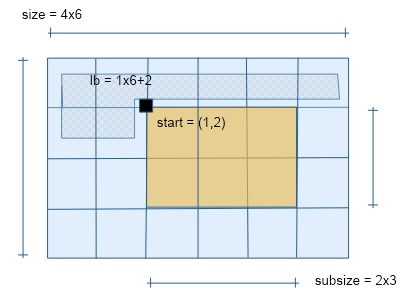FIGURE 6.12: True lower bound and extent of a subarray data type

The subarray datatype need not start at the first element of the buffer, so the extent is an overstatement of how much data is involved. In fact, the lower bound is zero, and the extent equals the size of the block from which the subarray is taken. The routine MPI_Type_get_true_extent returns the lower bound, indicating where the data starts, and the extent from that point. This is illustrated in figure  6.12  .

\csnippetwithoutput{trueextent}{examples/mpi/c}{trueextent}

There are also big data' routines MPI_Type_get_extent_x MPI_Type_get_true_extent_x that has an MPI_Count as output.

\begin{mpifournote} {Extent result as count} The C routines MPI_Type_get_extent_c MPI_Type_get_true_extent_c also output an MPI_Count  . \end{mpifournote}

### 6.6.2 Extent resizing

crumb trail: > mpi-data > Type extent > Extent resizing

A type is partly characterized by its lower bound and extent, or equivalently lower bound and upperbound. Somewhat miraculously, you can actually change these to achieve special effects. This is needed for:

• some cases of gather/scatter operations;

The problem here is that MPI uses the extent of the send type in a scatter, or the receive type in a gather: if that type is 20 bytes big from its first to its last element, then data will be read out 20 bytes apart in a scatter, or written 20 bytes apart in a gather. This ignores the gaps' in the type! (See exercise  6.8  .)

• When the count of derived items in a buffer is more than one.

Consider the vector type from the previous section. It is clear that

MPI_Type_vector( 2*count,bs,stride,oldtype,&two_n_type );

will not give the same result as
MPI_Type_vector( count,bs,stride,oldtype,&one_n_type );
MPI_Type_contiguous( 2,&one_n_type,&two_n_type );


The technicality on which the solution hinges is that you can resize' a type with MPI_Type_create_resized to give it a different extent, while not affecting how much data there actually is in it.

#### 6.6.2.1 Example 1

crumb trail: > mpi-data > Type extent > Extent resizing > Example 1

\includegraphics{extentpad} \caption{Contiguous type of two vectors, before and after resizing the extent.}

First consider sending more than one derived type, from a buffer containing consecutive integers:

// vectorpadsend.c
for (int i=0; i<max_elements; i++) sendbuffer[i] = i;
MPI_Type_vector(count,blocklength,stride,MPI_INT,&stridetype);
MPI_Type_commit(&stridetype);

We receive into a contiguous buffer:
MPI_Recv( recvbuffer,max_elements,MPI_INT, sender,0, comm,&status );
int count; MPI_Get_count(&status,MPI_INT,&count);
for (int i=0; i<count; i++) printf(" %d",recvbuffer[i]);
printf("\n");

giving an output of:
Receive 6 elements: 0 2 4 5 7 9

Next, we resize the type to ad the gap at the end. This is illustrated in figure  6.6.2.1  .

Resizing the type looks like:

MPI_Type_get_extent(stridetype,&l,&e);
printf("Stride type l=%ld e=%ld\n",l,e);
e += ( stride-blocklength) * sizeof(int);

and the corresponding output, including querying the extents, is:
Strided type l=0 e=20
Receive 6 elements: 0 2 4 6 8 10


#### 6.6.2.2 Example 2

crumb trail: > mpi-data > Type extent > Extent resizing > Example 2

For another example, let's revisit exercise  6.8 (and figure  6.8  ) where each process makes a buffer of integers that will be interleaved in a gather call: Strided data was sent in individual transactions. Would it be possible to address all these interleaved packets in one gather or scatter call?

int *mydata = (int*) malloc( localsize*sizeof(int) );
for (int i=0; i<localsize; i++)
mydata[i] = i*nprocs+procno;
MPI_Gather( mydata,localsize,MPI_INT,
/* rest to be determined */ );


An ordinary gather call will of course not interleave, but put the data end-to-end:

MPI_Gather( mydata,localsize,MPI_INT,
gathered,localsize,MPI_INT, // abutting
root,comm );

gather 4 elements from 3 procs:
0 3 6 9 1 4 7 10 2 5 8 11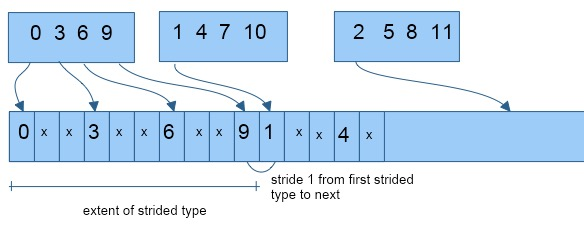FIGURE 6.13: Placement of gathered strided types.

Using a strided type still puts data end-to-end, but now there are unwritten gaps in the gather buffer:

MPI_Gather( mydata,localsize,MPI_INT,
gathered,1,stridetype, // abut with gaps
root,comm );

This is illustrated in figure  6.13  . A sample printout of the result would be:
 0 1879048192 1100361260 3 3 0 6 0 0 9 1 198654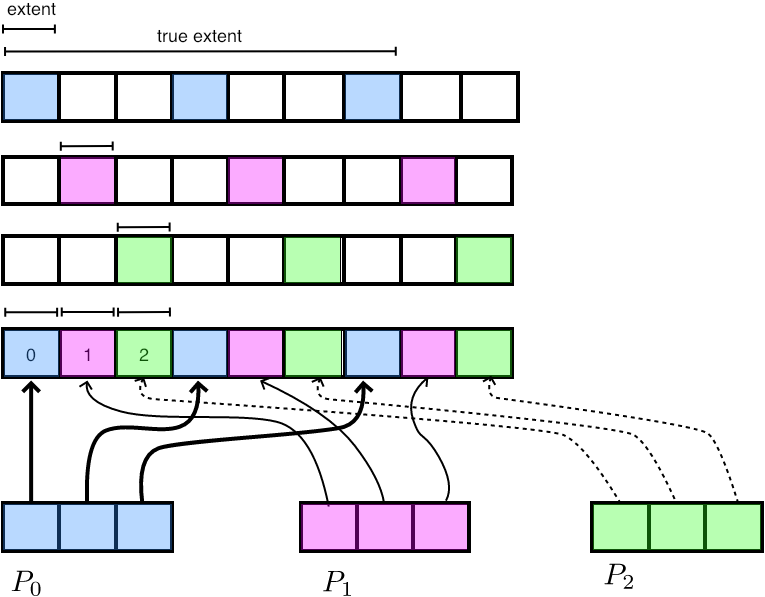FIGURE 6.14: Interleaved gather from data with resized extent

The trick is to use MPI_Type_create_resized to make the extent of the type only one int long:

// interleavegather.c
MPI_Datatype interleavetype;
MPI_Type_create_resized(stridetype,0,sizeof(int),&interleavetype);
MPI_Type_commit(&interleavetype);
MPI_Gather( mydata,localsize,MPI_INT,
gathered,1,interleavetype, // shrunk extent
root,comm );

Now data is written with the same stride, but at starting points equal to the shrunk extent:
 0 1 2 3 4 5 6 7 8 9 10 11


This is illustrated in figure  6.14  .

Exercise [stridesend] Rewrite exercise  6.8 to use a gather, rather than individual messages.
End of exercise

MPL note Resizing a datatype does not give a new type, but does the resize in place':

void layout::resize(ssize_t lb, ssize_t extent);

End of MPL note

#### 6.6.2.3 Example: dynamic vectors

crumb trail: > mpi-data > Type extent > Extent resizing > Example: dynamic vectors

Does it bother you (a little) that in the vector type you have to specify explicitly how many blocks there are? It would be nice if you could create a block with padding' and then send however many of those.

Well, you can introduce that padding by resizing a type, making it a little larger.

// stridestretch.c
MPI_Datatype oneblock;
MPI_Type_vector(1,1,stride,MPI_DOUBLE,&oneblock);
MPI_Type_commit(&oneblock);
MPI_Aint block_lb,block_x;
MPI_Type_get_extent(oneblock,&block_lb,&block_x);
printf("One block has extent: %ld\n",block_x);

// now send a bunch of these padded blocks


There is a second solution to this problem, using a structure type. This does not use resizing, but rather indicates a displacement that reaches to the end of the structure. We do this by putting a type MPI_UB at this displacement:

int blens; MPI_Aint displs;
blens = 1; blens = 1;
displs = 0; displs = 2 * sizeof(double);
types = MPI_DOUBLE; types = MPI_UB;
MPI_Status recv_status;


#### 6.6.2.4 Example: transpose

crumb trail: > mpi-data > Type extent > Extent resizing > Example: transpose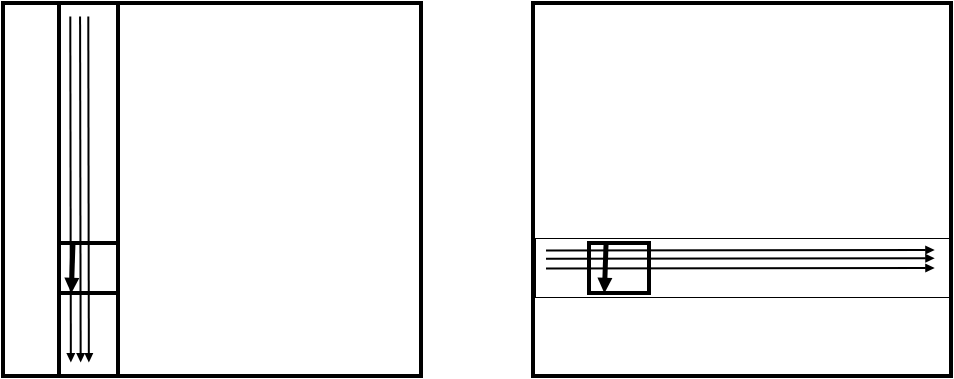FIGURE 6.15: Transposing a 1D partitioned array

Transposing data is an important part of such operations as the FFT  . We develop this in steps. Refer to figure  6.15  .

The source data can be described as a vector type defined as:

• there are $b$ blocks,
• of blocksize $b$,
• spaced apart by the global $i$-size of the array.
// transposeblock.cxx
MPI_Datatype sourceblock;
MPI_Type_vector( blocksize_j,blocksize_i,isize,MPI_INT,&sourceblock);
MPI_Type_commit( &sourceblock);


The target type is harder to describe. First we note that each contiguous block from the source type can be described as a vector type with:

• $b$ blocks,
• of size 1 each,
• stided by the global $j$-size of the matrix.
MPI_Datatype targetcolumn;
MPI_Type_vector( blocksize_i,1,jsize, MPI_INT,&targetcolumn);
MPI_Type_commit( &targetcolumn );


For the full type at the receiving process we now need to pack $b$ of these lines together.

Exercise Finish the code.

• What is the extent of the targetcolumn type?
• What is the spacing of the first elements of the blocks? How do you therefore resize the targetcolumn type?

End of exercise

## 6.7 Reconstructing types

crumb trail: > mpi-data > Reconstructing types

It is possible to find from a datatype how it was constructed. This uses the routines MPI_Type_get_envelope and MPI_Type_get_contents  . The first routine returns the combiner (with values such as MPI_COMBINER_VECTOR  ) and the number of parameters; the second routine is then used to retrieve the actual parameters.

## 6.8 Packing

crumb trail: > mpi-data > Packing

One of the reasons for derived datatypes is dealing with noncontiguous data. In older communication libraries this could only be done by packing data from its original containers into a buffer, and likewise unpacking it at the receiver into its destination data structures.

MPI offers this packing facility, partly for compatibility with such libraries, but also for reasons of flexibility. Unlike with derived datatypes, which transfers data atomically, packing routines add data sequentially to the buffer and unpacking takes them sequentially.

This means that one could pack an integer describing how many floating point numbers are in the rest of the packed message. Correspondingly, the unpack routine could then investigate the first integer and based on it unpack the right number of floating point numbers.

MPI offers the following:

• The MPI_Pack command adds data to a send buffer;
• the MPI_Unpack command retrieves data from a receive buffer;
• the buffer is sent with a datatype of MPI_PACKED  .

With MPI_Pack data elements can be added to a buffer one at a time. The position parameter is updated each time by the packing routine.

Conversely, MPI_Unpack retrieves one element from the buffer at a time. You need to specify the MPI datatype.

A packed buffer is sent or received with a datatype of MPI_PACKED  . The sending routine uses the position parameter to specify how much data is sent, but the receiving routine does not know this value a priori, so has to specify an upper bound.

\csnippetwithoutput{packunpack}{examples/mpi/c}{pack}

You can precompute the size of the required buffer with MPI_Pack_size  . \csnippetwithoutput{packsize}{examples/mpi/c}{packsize}

Exercise Suppose you have a structure of arrays'

struct aos {
int length;
double *reals;
double *imags;
};
`
with dynamically created arrays. Write code to send and receive this structure.
End of exercise

\newpage

## 6.9 Review questions

crumb trail: > mpi-data > Review questions

For all true/false questions, if you answer that a statement is false, give a one-line explanation.

1. Give two examples of MPI derived datatypes. What parameters are used to describe them?

2. Give a practical example where the sender uses a different type to send than the receiver uses in the corresponding receive call. Name the types involved.

3. Fortran only. True or false?
1. Array indices can be different between the send and receive buffer arrays.
2. It is allowed to send an array section.
3. You need to Reshape a multi-dimensional array to linear shape before you can send it.
4. An allocatable array, when dimensioned and allocated, is treated by MPI as if it were a normal static array, when used as send buffer.
5. An allocatable array is allocated if you use it as the receive buffer: it is filled with the incoming data.
4. Fortran only: how do you handle the case where you want to use an allocatable array as receive buffer, but it has not been allocated yet, and you do not know the size of the incoming data?